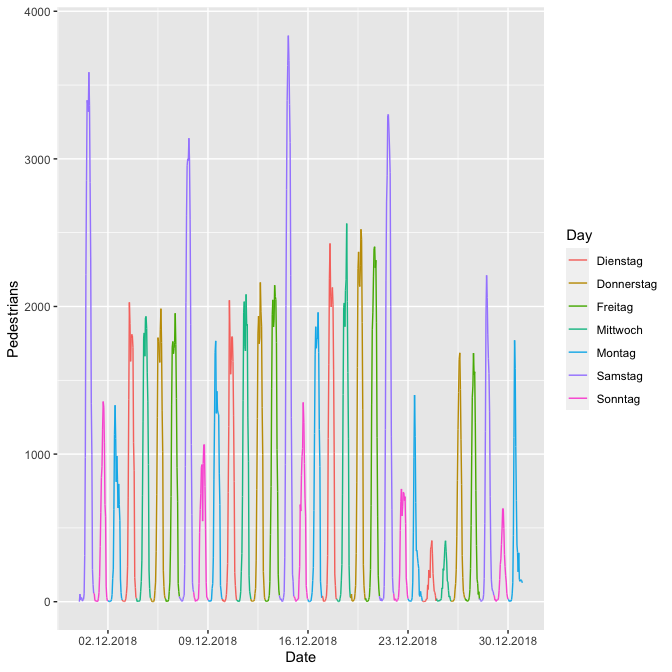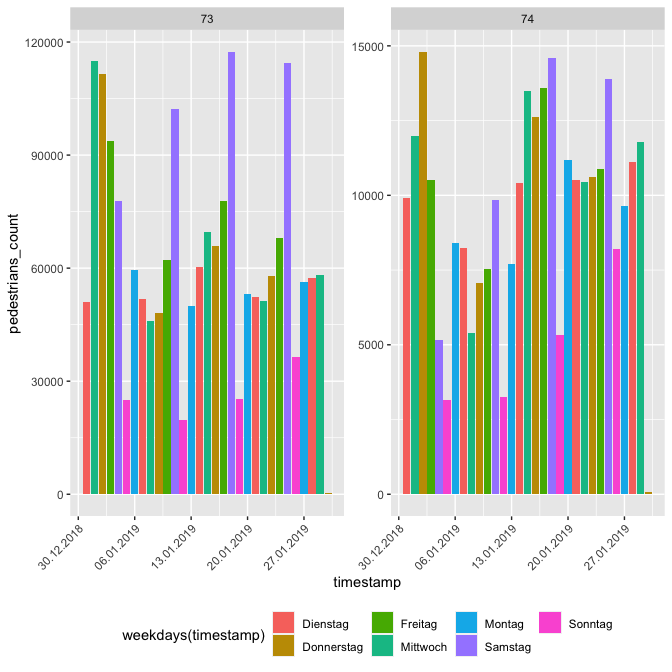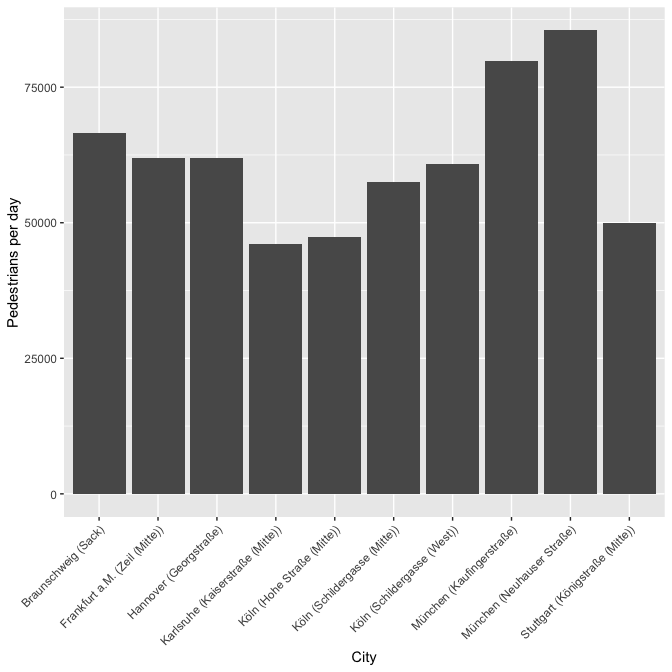# 2 Installation

Until now the package is not on CRAN but you can download it via GitHub with the following command:

# 3 API Keys

To use this package, you will first need to get your hystreet API key. To do so, go to this link: https://hystreet.com/

Now you have three options:

Once you have your key, save it as an environment variable for the current session by running the following:

Sys.setenv(HYSTREET_API_TOKEN = "PASTE YOUR API TOKEN HERE")
1. Alternatively, you can set it permanently with the help of usethis::edit_r_environ() by adding the line to your .Renviron:
HYSTREET_API_TOKEN = PASTE YOUR API TOKEN HERE
1. If you don’t want to save it here, you can input it in each function using the API_token parameter.

# 4 Usage

Function name Description Example
get_hystreet_stats() request common statistics about the hystreet project get_hystreet_stats()
get_hystreet_locations() request all qvailable locations get_hystreet_locations()
get_hystreet_station_data() request data from a stations get_hystreet_station_data(71)
set_hystreet_token() set your API token set_hystreet_token(123456789)

The function ‘get_hystreet_stats()’ summarises the number of available stations and the sum of all counted pedestrians.

library(hystReet)

stats <- get_hystreet_stats()

## 4.1 Request all stations

The function ‘get_hystreet_locations()’ requests all available stations of the project.

locations <- get_hystreet_locations()
id name city
251 Annastraße Augsburg
209 Lange Straße Oldenburg
151 Stiftstraße Stuttgart
253 Neustraße Bocholt
168 Hohe Straße (Nord) Köln
93 Große Bleichen Hamburg
135 Schönbornstraße Würzburg
252 Holm Flensburg
131 Sack Braunschweig
142 Hauptstraße (Süd) Erlangen

## 4.2 Request data from a specific station

The (probably) most interesting function is ‘get_hystreet_station_data()’. With the hystreetID it is possible to request a specific station. By default, all the data from the current day are received. With the ‘query’ argument it is possible to set the received data more precise: * from: datetime of earliest measurement (default: today 00:00:00:): e.g. “2018-10-01 12:00:00” or “2018-10-01” * to : datetime of latest measurement (default: today 23:59:59): e.g. “2018-12-01 12:00:00” or “2018-12-01” * resoution: Resultion for the measurement grouping (default: hour): “day”, “hour”, “month”, “week”

location_71 <- get_hystreet_station_data(
hystreetId = 71,
query = list(from = "2018-12-01", to = "2018-12-31", resolution = "day"))

# 5 Some ideas to visualise the data

Let´s see if we can see the most frequent days before christmas … I think it could be saturday ;-). Also nice to see the 24th and 25th of December … holidays in Germany :-).

location_71 <- get_hystreet_station_data(
hystreetId = 71,
query = list(from = "2018-12-01", to = "2019-01-01", resolution = "hour"))
ggplot(location_71$measurements, aes(x = timestamp, y = pedestrians_count, colour = weekdays(timestamp))) + geom_path(group = 1) + scale_x_datetime(date_breaks = "7 days") + scale_x_datetime(labels = date_format("%d.%m.%Y")) + labs(x = "Date", y = "Pedestrians", colour = "Day") ## Scale for 'x' is already present. Adding another scale for 'x', which will ## replace the existing scale.## 5.1 Compare different stations Now let´s compare different stations: 1. Load the data location_73 <- get_hystreet_station_data( hystreetId = 73, query = list(from = "2019-01-01", to = "2019-01-31", resolution = "day"))$measurements %>%
select(pedestrians_count, timestamp) %>%
mutate(station = 73)

location_74 <- get_hystreet_station_data(
hystreetId = 74,
query = list(from = "2019-01-01", to = "2019-01-31", resolution = "day"))$measurements %>% select(pedestrians_count, timestamp) %>% mutate(station = 74) data <- bind_rows(location_73, location_74) ggplot(data, aes(x = timestamp, y = pedestrians_count, fill = weekdays(timestamp))) + geom_bar(stat = "identity") + scale_x_datetime(labels = date_format("%d.%m.%Y")) + facet_wrap(~station, scales = "free_y") + theme(legend.position = "bottom", axis.text.x = element_text(angle = 45, hjust = 1))## 5.2 Highest ratio (pedestrians/day) Now a little bit of big data analysis. Let´s find the station with the highest pedestrians per day ratio: hystreet_ids <- get_hystreet_locations() all_data <- lapply(hystreet_ids[,"id"], function(x){ temp <- get_hystreet_station_data( hystreetId = x) lifetime_count <- temp$statistics$lifetime_count days_counted <- as.numeric(temp$metadata$latest_measurement_at - temp$metadata$earliest_measurement_at) return(data.frame( id = x, station = paste0(temp$city, " (",temp\$name,")"),

})

ratio <- bind_rows(all_data)

What stations have the highest ratio?

ratio %>%
top_n(5, ratio) %>%
arrange(desc(ratio))
##    id                       station    ratio
## 1  73    München (Neuhauser Straße) 85458.05
## 2 165     München (Kaufingerstraße) 79790.84
## 3 131           Braunschweig (Sack) 66558.89
## 4 150 Frankfurt a.M. (Zeil (Mitte)) 61987.19
## 5  63        Hannover (Georgstraße) 61943.64

Now let´s visualise the top 10 cities:

ggplot(ratio %>%
top_n(10,ratio), aes(station, ratio)) +
geom_bar(stat = "identity") +
labs(x = "City",
y = "Pedestrians per day") +
theme(legend.position = "bottom",
axis.text.x = element_text(angle = 45, hjust = 1))